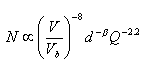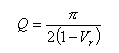NESS ENGINEERING
TECHNICAL DATA

High voltage capacitor lifetime is generally a very strong function of the operating voltage. In addition, the voltage reversal applied to the capacitor can also influence the lifetime.

For plastic film capacitors, the lifetime of a capacitor in terms of N (number of discharges) is generally as follows:Where:

N = number of discharges to failure

V = application operating voltage

Vb = capacitor breakdown voltage

d = dielectric thickness

b = thickness exponent, typically less than 3

Q = circuit quality factor

The circuit quality factor is generally related to the voltage reversal as follows:Where:

Vr = voltage reversal (0 < Vr < 1 so that a voltage reversal of 50% yields a Vr = 0.5)

For ceramic dielectric capacitors, the lifetime of a capacitor is generally as follows:Send consulting inquiries, comments, and suggestions to nessengr@san.rr.com

Ness Engineering, Inc.
P.O. Box 261501
San Diego, CA 92196
(858) 566-2372
(858) 240-2299 FAX

 © Richard M. Ness and Ness Engineering, Inc. Last Updated: